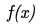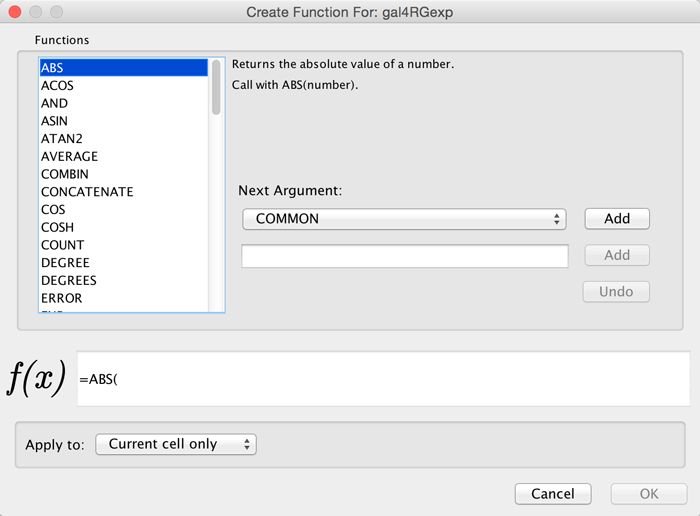# 9. Column Data Functions and Equations¶

## 9.1. Column Formulas¶

### Introduction¶

Column data values may be formulas. A typical example is =ABS(\$otherColumn + LOG(10.2)). Formulas are modeled after Excel(tm) but only support references to other columns at the same node, edge or network. Since Cytoscape column names may contain embedded spaces, optional braces around the column name (required if the name is not simply a letter followed by one or more letters or digits) is allowed e.g. \${a name with spaces}. Backslashes, opening braces and dollar signs in column names have to be escaped with a leading backslash. For example the column name ex\$am{p\le would have to be written as \${ex\\$am\{p\\le}. Finally, column names are case sensitive.

String constants are written with double-quotes . In order to embed a double-quote or a backslash in a string they have to be escaped with a leading backslash, therefore the string “\ must be written as “\”\\”. Formula results must be compatible with the type of the column that they have been assigned to. The rules are rather lax though, for example anything can be interpreted as a string and all numeric values will be accepted for a boolean (or logical) column data where non-zero will be interpreted as true and zero as false. For integer columns, floating point values will be converted using the rules of the Excel(tm) INT function. Parentheses can be used for grouping and to change evaluation order. The operator precedence rules follow those of standard arithmetic.

### Operators¶

Currently supported operators are the four basic arithmetic operators and the ^ exponentiation operator. +, -, *, and \ are left-associative and ^ is right-associative. The string concatenation operator is &. Supported boolean or logical operators are the comparison operators <, >, <=, >=, =, and <> (not equal).

### Supported Functions¶

Currently we support the following functions:

#### Cytoscape-specific functions¶

• Degree – the degree of a node.
• InDegree – the indegree of a node.
• OutDegree – the outdegree of a node.
• SourceID – the ID of the source node of an edge.
• TargetID – the ID of the target of an edge.

#### Numeric Functions¶

• Abs – Returns the absolute value of a number.
• ACos – Returns the arccosine of a number.
• ASin – Returns the arcsine of a number.
• ATan2 – Returns the arctangent of two numbers x and y.
• Average – Returns the average of a group of numbers.
• Cos – Returns the cosine of an angle given in radians.
• Cosh – Returns the hyperbolic sine of its argument.
• Count – Returns the number of numeric values in a list.
• Degrees – Returns its argument converted from radians to degrees.
• Exp – Returns e raised to a specified number.
• Ln – Returns the natural logarithm of a number.
• Log – Returns the logarithm of a number to a specified base.
• Max – Returns the maximum of a group of numbers.
• Median – Returns the median of a list of numbers.
• Min – Returns the minimum of a group of numbers.
• Mod – Calculates the modulus of a number.
• Pi – Returns an approximation of the value of p.
• Round – Rounds a number to a specified number of decimal places.
• Sin – Returns the sine of an angle given in radians.
• Sinh – Returns the hyperbolic sine of its argument.
• Sqrt – Calculates the square root of a number.
• Tan – returns the tangent of its argument in radians.
• Tanh – returns the hyperbolic tangent of its argument in radians.
• Trunc – Truncates a number.

#### String Functions¶

• Concatenate – Concatenates two or more pieces of text.
• Left – Returns a prefix of s string.
• Len – Returns the length of a string.
• Lower – Converts a string to lowercase.
• Mid – Selects a substring of some text.
• Right – Returns a suffix of a string.
• Substitute – Replaces some text with other text.
• Text – Format a number using the Java DecimalFormat class’ conventions.
• Upper – Converts a string to uppercase.
• Value – Converts a string to a number.

#### Logical/Boolean Functions¶

• And – Returns the logical conjunction of any number of boolean values.
• Not – Returns the logical negation of a boolean value.
• Or – Returns the logical disjunction of any number of boolean values.

#### List Functions¶

• First – Returns the first entry in a list.
• Last – Returns the last entry in a list.
• Nth – Returns the n-th entry in a list.

#### Statistical Functions¶

• Largest – the kth largest value in a list.
• GeoMean – the geometric mean of a set of numbers.
• HarMean – the harmonic mean of a set of numbers.
• Mode – the mode of a set of numbers.
• NormDist – Returns the pdf or CDF of the normal distribution.
• Permut – Returns the number of permutations for a given number of objects.
• StDev - sample standard deviation.
• Var – sample variance.

#### Miscellaneous Functions¶

• Combin - Returns the number of combinations for a given number of objects.
• If – Returns one of two alternatives based on a boolean value.
• ListToString – Returns a string representation of a list.
• Now – Returns a string representation of the current date and time.
• Today – returns a string representation of the current date.

### Pitfalls¶

The possibly biggest problem is the referencing of other columns that have null values. This is not allowed and leads to errors. In order to mitigate this problem we support the following optional syntax for column references: \${columnName:defaultValue}. The interpretation is that if columnName is null, then the default value will be used, otherwise the value of the referenced value will be used instead. The referenced column must still be a defined column and not an arbitrary name! The other potential problem is when there are circular column reference dependencies. Circular dependencies will be detected at formula evaluation time and lead to a run-time error.

### Useful Tips¶

When working with formulas it can be very helpful to open the Developer’s Log Console. Formula evaluation errors will be logged there.

## 9.2. The Formula Builder¶

In order to ease the creation of formulas as well as to facilitate discovery of built-in functions we provide a Function Builder in the Table Panel. After selecting a non-list column cell, you can invoke it by clicking onfx-button.png. This should bring up the Function Builder which looks like this:FunctionBuilder2.png

Select a function on the left hand side of the dialog - here, we’ve selected the ABS function. Next to the list of functions, you can specify one or more arguments. This can either be a column (selected from the drop-down list) or a constant specified in the box below. If you select a column, the value of that column (in the row containing the formula) will be used, and the function result will be updated dynamically when that value changes. Click Add to add an argument - you can add one or more depending on how many arguments the function accepts. At the bottom of the dialog is a preview of the current formula. Under Apply to, you can select whether the formula will apply to the current cell only, the cell selection, or the entire column. Click OK when you are satisfied with the result, or Cancel to discard any changes.

The Function Builder is a useful tool for discovery of the list of built-in functions, which has the return type matching the data type of the column. Arguments can either be selected from a list of named columns, or constant values can be entered in a text entry field. A major shortcoming at this time is that the Formula Builder won’t let you compose functions with function calls as arguments. If you need the most general functionality, please type the expression directly into a cell.

## 9.3. A Note for App Writers¶

It is relatively easy to add your own built-in formula functions. A simple function can probably be implemented in 15 to 20 minutes. It can then be registered via the parser and becomes immediately available to the user. It will of course also show up in the drop-down list in the Function Builder.